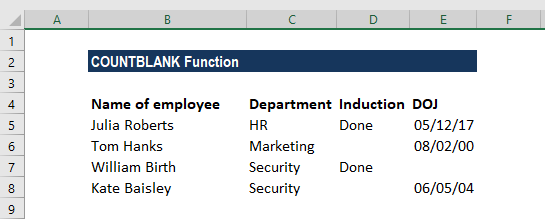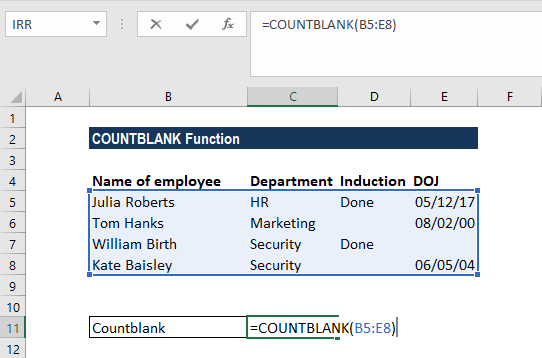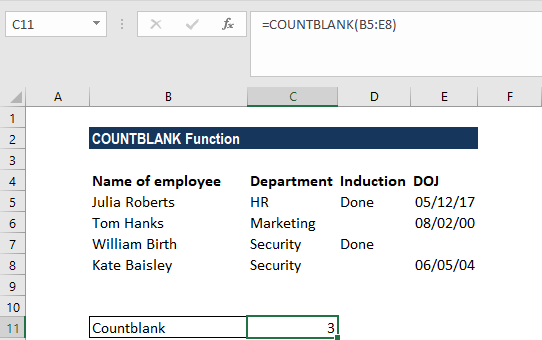# COUNTBLANK Function

Counts the number of empty cells in a given range of cells

## What is the COUNTBLANK Function?

The COUNTBLANK function is categorized under Excel STATISTICAL functions. COUNTBLANK will count the number of empty cells in a given range of cells.

In financial analysis, the function can be useful in highlighting or counting empty cells in a given range.

### Formula

=COUNTBLANK(range)

Where:

1. Range specifies the range of cells in which we want to count blank cells.

For the COUNTBLANK function, remember that:

• Any cells that contain text, numbers, errors, etc. are not counted by this function.
• Formulas that return empty text (“”) are considered blank and will be counted. So, if a cell contains an empty text string or a formula that returns an empty text string, the cell is counted as blank by the COUNTBLANK function.
• Cells that contain zero are considered not blank and will not be counted.

### How to use the COUNTBLANK Function in Excel?

As a worksheet function, COUNTBLANK can be entered as part of a formula in a cell of a worksheet. To understand the uses of the function, let us consider an example:

#### Worked Example of COUNTBLANK

If we wish to know the number of cells that are empty, we can use conditional formatting with this function.

Suppose we are given the data below:Here, we can use the formula =COUNTBLANK(A2:D5) to count the empty rows:We get the results below:If we want, we can use conditional formatting to highlight rows with empty cells using the COUNTBLANK function. Select the desired range, and on the select conditional formatting and apply the COUNTBLANK() function. This will then highlight all the cells that are Blank on the desired range.

Thanks for reading CFI’s guide to important Excel functions! By taking the time to learn and master these functions, you’ll significantly speed up your financial modeling and analysis. To learn more, check out these additional CFI resources:

• Free Excel Course
• Excel Formulas for Advanced Users
• Excel Shortcut Keys
• Financial Modeling Guide

### Free Excel Tutorial

To master the art of Excel, check out CFI’s FREE Excel Crash Course, which teaches you how to become an Excel power user.  Learn the most important formulas, functions, and shortcuts to become confident in your financial analysis.

Launch CFI’s Free Excel Course now to take your career to the next level and move up the ladder!